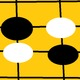# Unresolved Detail In Plotted Equations

Sometimes the calculator detects that an equation is too complicated to plot perfectly in a reasonable amount of time. When this happens, the equation is plotted at lower resolution.

267 out of 297 found this helpful
Have more questions? Submit a request

•22yatzac

x^a+y^a=1

when a is less than -1

•Thurston Domina

\sin\left(\frac{x}{y}\right)=\cos\left(x\cdot y\right)

•Thurston Domina
•Thurston Domina
Edited by Thurston Domina
•Thurston Domina
•Thurston Domina
•Thurston Domina
•Thurston Domina
•mATT C
•Tanksear Industries

\tan\left(\log\left(\csc\left(\operatorname{floor}\left(\cot\left(x\right)\right)\right)\right)\right)=\operatorname{mod}\left(x,x\right)

It says it, but if you can find the lines themselves, I commend you.

*You need to zoom out a couple times*

Edited by Tanksear Industries
•mATT C

@Tanksear Industries

I see them.

•Tanksear Industries

Where? Maybe I didn't look in the right place (or maybe my computer can't render it)

Edited by Tanksear Industries
•mATT C

@Tanksear Industries•mATT C
•22yatzac

\sin xx=\cos2y

•Zombie Chicken

y = y!/x!

•GlitchedGraph666

Infinite \sin\left(x+y^x-x^2\right)=\cos\left(xy+y^2\right)

•GlitchedGraph666

\sin\left(x^2+y^2-x+\sin\left(x^2\right)+\tan\left(x^{\sin\left(y\right)}\right)\right)=\cos\left(x^2\right)

\sin\left(x^2\right)\cdot\sqrt{\pi+\sin\left(x^2\right)}+y\cdot\sin\left(x^y+x^{\sin\left(y\right)}\right)=\cos\left(x^{\cos\left(x\right)}+y\right)

\log\left(x^y\right)\cdot\sin\left(x^{\sin\left(y\right)}-\cos\left(x^2\right)\right)=\sin\left(x^{\sin\left(x\right)}\right)+\sin\left(y\right)

\sin\left(x^{\cos\left(y\right)}+x^2-y^2\cdot\frac{x}{y^2}\right)=\cos\left(x^{\sin\left(y\right)}\right)

\tan\left(x\cdot y^{\sin\left(x\right)}\right)=\sin\left(x!y!\right)\cdot\sin\left(x+y\right)

\ln\left(x!\right)\cdot y\sin\left(x^y\right)=\sin\left(x^{\cos\left(y\right)}\right)

\cos\left(x^{\sin\left(y\right)}\cdot\log\left(x^y\right)\right)=\cos\left(\ln\left(x\cdot y^x\right)\right)

\sin\left(x\cdot\cos\left(y^2\right)+x^2\right)=\sin\left(\ln\left(x^y\right)\right)

\sin\left(yx^2\right)=\sin\left(y^2+x^2\right)+\tan\left(y^2\right)

Combine all of them for death!!!

https://www.desmos.com/calculator/08pvl7xy1d

•Zapyourtumor3

Even though this graph seems like there is unresolved detail, it doesn't say so:

https://www.desmos.com/calculator/iwi50btnpd

•22yatzac

Tupper's self-referential formula

\frac{1}{2}<\left|\operatorname{mod}\left(2^{\left(-17\left|x\right|-\operatorname{mod}\left(y,17\right)\right)}\left|\frac{y}{17}\right|,2\right)\right|

•Harry Lennox

\frac{\cos x}{\sin y}=\cos x^y

•Harry Lennox

\sin\left(x!+y!\right)=0

•Harry Lennox

\frac{\sin\left(xy\right)+\cos\left(xy\right)+\tan\left(xy\right)+\sec\left(xy\right)+\csc\left(xy\right)+\cot\left(xy\right)}{\frac{e^{\sin x}}{\frac{e^{\cos x}}{e^{\tan x}}}}=3

\frac{x!}{y!}=0

\frac{x^{\frac{e!}{y!}}}{\sin\left(\frac{x!}{y!^{e!}}\right)}=0

y!<1

y!x!<1

\frac{\left(\sin\left(x\right)\cos\left(x\right)\tan\left(x\right)\sec\left(x\right)\csc\left(x\right)\cot\left(x\right)\right)^{x!^{y!}}}{\frac{x!}{y!}}\ge x!y!

\operatorname{mod}\left(\sin\left(x^3\right),\sin\left(y^3\right)\right)=\operatorname{floor}\left(\sin x\right)

•Javauser101

x^{\frac{\cos\left(\frac{x^2}{y^2}\right)}{\tan\left(\frac{x^2}{y^2}\right)}}=x^2\cdot y^2

•Harry Lennox

\frac{\operatorname{floor}\left(x\right)}{\operatorname{floor}\left(e^{\sin\left(x\right)\cos\left(y\right)}\right)}=\operatorname{floor}\left(x\right) is very interesting.

•TheRath27

y=\sec xy

•William Gross

type in these four IN THIS ORDER on one graph

and watch as you see the entire universe explode

also you computer might possibly puke on you :)             so be careful

tan(y)^2=sin(x)^2

sin(y)^2=cos(x)^2

tan(x/y)=x

sin(x^2)=cos(y^2)

•Thomashill

y\cdot x=\frac{x^2}{1y}+\frac{12^{2^y}\pi^{yx}}{yxy}

•Tyler Hil

•